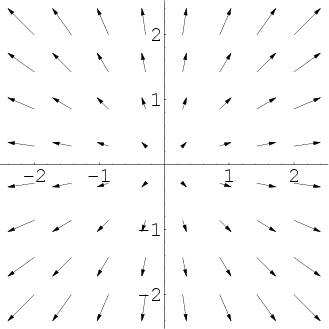# Math Insight

### Image: A 2D vector field pointing outwardThis vector field illustrates expansion or positive divergence. The field is $\dlvf(x,y)=(x,y)$. Its divergence is $\div \vc{\dlvf}=\pdiff{\dlvfc_1}{x}+\pdiff{\dlvfc_2}{y}=1+1=2$.

Image file: vector_field_explosion.png